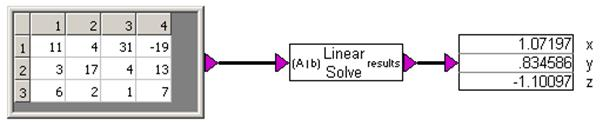## linearSolve

Block Category: Matrix Operation

Description: The linearSolve block solves systems of linear equations of the A * X = b, where A is an N-by-N matrix and X and b are N-by-1 matrices. This block will also compute the least squares solution to an over-determined system, or the minimum norm solution to an under-determined system.

The linearSolve block accepts as input an extended matrix (A | b). That is, if A is N-by-N and b is N-by-1, then the input matrix must be N-by- (N + 1) and the last column is of components of b.

#### Example

The linearSolve block computes the values of three unknowns (x, y, and z) in the following three equations:

11x + 4y + 31x = -19

3x + 17y + 4z = 13

6x + 2y + 1z = 7